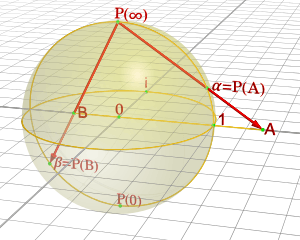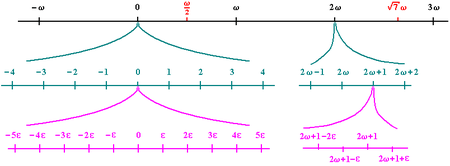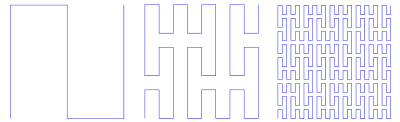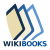# Infinity

For other uses of "Infinity" and "Infinite", see Infinity (disambiguation).Infinity (symbol: ) is an abstract concept describing something without any bound or larger than any number. Philosophers have speculated about the nature of the infinite, such as Zeno of Elea, who proposed many paradoxes involving infinity, and Eudoxus of Cnidus, who used the idea of infinitely small quantities in his method of exhaustion. Modern mathematics uses the concept of infinity in the solution of many practical and theoretical problems, such as in calculus and set theory, and the idea also is used in physics and the other sciences.

In mathematics, "infinity" is often treated as a number (i.e., it counts or measures things: "an infinite number of terms") but it is not the same sort of number as natural or real numbers.

Georg Cantor formalized many ideas related to infinity and infinite sets during the late 19th and early 20th centuries. In the theory he developed, there are infinite sets of different sizes (called cardinalities). For example, the set of integers is countably infinite, while the infinite set of real numbers is uncountable.

## History

Main article: Infinity (philosophy)

Ancient cultures had various ideas about the nature of infinity. The ancient Indians and Greeks did not define infinity in precise formalism as does modern mathematics, and instead approached infinity as a philosophical concept.

### Early Greek

The earliest recorded idea of infinity comes from Anaximander, a pre-Socratic Greek philosopher who lived in Miletus. He used the word apeiron which means infinite or limitless. However, the earliest attestable accounts of mathematical infinity come from Zeno of Elea (c. 490 BCE? – c. 430 BCE?), a pre-Socratic Greek philosopher of southern Italy and member of the Eleatic School founded by Parmenides. Aristotle called him the inventor of the dialectic. He is best known for his paradoxes, described by Bertrand Russell as "immeasurably subtle and profound".

In accordance with the traditional view of Aristotle, the Hellenistic Greeks generally preferred to distinguish the potential infinity from the actual infinity; for example, instead of saying that there are an infinity of primes, Euclid prefers instead to say that there are more prime numbers than contained in any given collection of prime numbers (Elements, Book IX, Proposition 20).

However, recent readings of the Archimedes Palimpsest have hinted that Archimedes at least had an intuition about actual infinite quantities.

### Early Indian

The Indian mathematical text Surya Prajnapti (c. 4th–3rd century BCE) classifies all numbers into three sets: enumerable, innumerable, and infinite. Each of these was further subdivided into three orders:

• Enumerable: lowest, intermediate, and highest
• Innumerable: nearly innumerable, truly innumerable, and innumerably innumerable
• Infinite: nearly infinite, truly infinite, infinitely infinite

In this work, two basic types of infinite numbers are distinguished. On both physical and ontological grounds, a distinction was made between asaṃkhyāta ("countless, innumerable") and ananta ("endless, unlimited"), between rigidly bounded and loosely bounded infinities.

### 17th century

European mathematicians started using infinite numbers in a systematic fashion in the 17th century. John Wallis first used the notationfor such a number, and exploited it in area calculations by dividing the region into infinitesimal strips of width on the order of. Euler used the notationfor an infinite number, and exploited it by applying the binomial formula to the'th power, and infinite products offactors.

In 1699 Isaac Newton wrote about equations with an infinite number of terms in his work De analysi per aequationes numero terminorum infinitas.

## Mathematics

### Infinity symbol

Main article: Infinity symbol

The infinity symbol(sometimes called the lemniscate) is a mathematical symbol representing the concept of infinity. The symbol is encoded in Unicode at U+221E INFINITY (HTML &#8734; · &infin;) and in LaTeX as \infty.

It was introduced in 1655 by John Wallis, and, since its introduction, has also been used outside mathematics in modern mysticism and literary symbology.

### Calculus

Leibniz, one of the co-inventors of infinitesimal calculus, speculated widely about infinite numbers and their use in mathematics. To Leibniz, both infinitesimals and infinite quantities were ideal entities, not of the same nature as appreciable quantities, but enjoying the same properties in accordance with the Law of Continuity.

#### Real analysis

In real analysis, the symbol, called "infinity", is used to denote an unbounded limit.means that x grows without bound, andmeans the value of x is decreasing without bound. If f(t) ≥ 0 for every t, then

•means that f(t) does not bound a finite area fromto•means that the area under f(t) is infinite.
•means that the total area under f(t) is finite, and equalsInfinity is also used to describe infinite series:

•means that the sum of the infinite series converges to some real value.
•means that the sum of the infinite series diverges in the specific sense that the partial sums grow without bound.

Infinity can be used not only to define a limit but as a value in the extended real number system. Points labeledandcan be added to the topological space of the real numbers, producing the two-point compactification of the real numbers. Adding algebraic properties to this gives us the extended real numbers. We can also treatandas the same, leading to the one-point compactification of the real numbers, which is the real projective line. Projective geometry also refers to a line at infinity in plane geometry, a plane at infinity in three-dimensional space, and so forth for higher dimensions.

#### Complex analysisBy stereographic projection, the complex plane can be "wrapped" onto a sphere, with the top point of the sphere corresponding to infinity. This is called the Riemann sphere.

In complex analysis the symbol, called "infinity", denotes an unsigned infinite limit.means that the magnitudeof x grows beyond any assigned value. A point labeledcan be added to the complex plane as a topological space giving the one-point compactification of the complex plane. When this is done, the resulting space is a one-dimensional complex manifold, or Riemann surface, called the extended complex plane or the Riemann sphere. Arithmetic operations similar to those given above for the extended real numbers can also be defined, though there is no distinction in the signs (therefore one exception is that infinity cannot be added to itself). On the other hand, this kind of infinity enables division by zero, namelyfor any nonzero complex number z. In this context it is often useful to consider meromorphic functions as maps into the Riemann sphere taking the value ofat the poles. The domain of a complex-valued function may be extended to include the point at infinity as well. One important example of such functions is the group of Möbius transformations.

### Nonstandard analysisInfinitesimals (ε) and infinites (ω) on the hyperreal number line (1/ε = ω/1)

The original formulation of infinitesimal calculus by Isaac Newton and Gottfried Leibniz used infinitesimal quantities. In the twentieth century, it was shown that this treatment could be put on a rigorous footing through various logical systems, including smooth infinitesimal analysis and nonstandard analysis. In the latter, infinitesimals are invertible, and their inverses are infinite numbers. The infinities in this sense are part of a hyperreal field; there is no equivalence between them as with the Cantorian transfinites. For example, if H is an infinite number, then H + H = 2H and H + 1 are distinct infinite numbers. This approach to non-standard calculus is fully developed in Keisler (1986).

### Set theory

Main articles: Cardinality and Ordinal numberOne-to-one correspondence between infinite set and proper subset

A different form of "infinity" are the ordinal and cardinal infinities of set theory. Georg Cantor developed a system of transfinite numbers, in which the first transfinite cardinal is aleph-null (0), the cardinality of the set of natural numbers. This modern mathematical conception of the quantitative infinite developed in the late nineteenth century from work by Cantor, Gottlob Frege, Richard Dedekind and others, using the idea of collections, or sets.

Dedekind's approach was essentially to adopt the idea of one-to-one correspondence as a standard for comparing the size of sets, and to reject the view of Galileo (which derived from Euclid) that the whole cannot be the same size as the part (however, see Galileo's paradox where he concludes that positive integers which are squares and all positive integers are the same size). An infinite set can simply be defined as one having the same size as at least one of its proper parts; this notion of infinity is called Dedekind infinite. The diagram gives an example: viewing lines as infinite sets of points, the left half of the lower blue line can be mapped in a one-to-one manner (green correspondences) to the higher blue line, and, in turn, to the whole lower blue line (red correspondences); therefore the whole lower blue line and its left half have the same cardinality, i.e. "size".

Cantor defined two kinds of infinite numbers: ordinal numbers and cardinal numbers. Ordinal numbers may be identified with well-ordered sets, or counting carried on to any stopping point, including points after an infinite number have already been counted. Generalizing finite and the ordinary infinite sequences which are maps from the positive integers leads to mappings from ordinal numbers, and transfinite sequences. Cardinal numbers define the size of sets, meaning how many members they contain, and can be standardized by choosing the first ordinal number of a certain size to represent the cardinal number of that size. The smallest ordinal infinity is that of the positive integers, and any set which has the cardinality of the integers is countably infinite. If a set is too large to be put in one to one correspondence with the positive integers, it is called uncountable. Cantor's views prevailed and modern mathematics accepts actual infinity. Certain extended number systems, such as the hyperreal numbers, incorporate the ordinary (finite) numbers and infinite numbers of different sizes.

#### Cardinality of the continuum

One of Cantor's most important results was that the cardinality of the continuumis greater than that of the natural numbers; that is, there are more real numbers R than natural numbers N. Namely, Cantor showed that(see Cantor's diagonal argument or Cantor's first uncountability proof).

The continuum hypothesis states that there is no cardinal number between the cardinality of the reals and the cardinality of the natural numbers, that is,(see Beth one). However, this hypothesis can neither be proved nor disproved within the widely accepted Zermelo–Fraenkel set theory, even assuming the Axiom of Choice.

Cardinal arithmetic can be used to show not only that the number of points in a real number line is equal to the number of points in any segment of that line, but that this is equal to the number of points on a plane and, indeed, in any finite-dimensional space.The first three steps of a fractal construction whose limit is a space-filling curve, showing that there are as many points in a one-dimensional line as in a two-dimensional square.

The first of these results is apparent by considering, for instance, the tangent function, which provides a one-to-one correspondence between the interval (−π/2, π/2) and R (see also Hilbert's paradox of the Grand Hotel). The second result was proved by Cantor in 1878, but only became intuitively apparent in 1890, when Giuseppe Peano introduced the space-filling curves, curved lines that twist and turn enough to fill the whole of any square, or cube, or hypercube, or finite-dimensional space. These curves can be used to define a one-to-one correspondence between the points in the side of a square and those in the square.

### Geometry and topology

Infinite-dimensional spaces are widely used in geometry and topology, particularly as classifying spaces, such as Eilenberg−MacLane spaces. Common examples are the infinite-dimensional complex projective space K(Z,2) and the infinite-dimensional real projective space K(Z/2Z,1).

### Fractals

The structure of a fractal object is reiterated in its magnifications. Fractals can be magnified indefinitely without losing their structure and becoming "smooth"; they have infinite perimeters—some with infinite, and others with finite surface areas. One such fractal curve with an infinite perimeter and finite surface area is the Koch snowflake.

### Mathematics without infinity

Leopold Kronecker was skeptical of the notion of infinity and how his fellow mathematicians were using it in the 1870s and 1880s. This skepticism was developed in the philosophy of mathematics called finitism, an extreme form of the philosophical and mathematical schools of constructivism and intuitionism.

## Physics

In physics, approximations of real numbers are used for continuous measurements and natural numbers are used for discrete measurements (i.e. counting). It is therefore assumed by physicists that no measurable quantity could have an infinite value . For instance, by taking an infinite value in an extended real number system, or by requiring the counting of an infinite number of events. It is, for example, presumed impossible for any type of body to have infinite mass or infinite energy. Concepts of infinite things such as an infinite plane wave exist, but there are no experimental means to generate them.

### Theoretical applications of physical infinity

The practice of refusing infinite values for measurable quantities does not come from a priori or ideological motivations, but rather from more methodological and pragmatic motivations . One of the needs of any physical and scientific theory is to give usable formulas that correspond to or at least approximate reality. As an example, if any object of infinite gravitational mass were to exist, any usage of the formula to calculate the gravitational force would lead to an infinite result, which would be of no benefit since the result would be always the same regardless of the position and the mass of the other object. The formula would be useful neither to compute the force between two objects of finite mass nor to compute their motions. If an infinite mass object were to exist, any object of finite mass would be attracted with infinite force (and hence acceleration) by the infinite mass object, which is not what we can observe in reality. Sometimes infinite result of a physical quantity may mean that the theory being used to compute the result may be approaching the point where it fails. This may help to indicate the limitations of a theory.

This point of view does not mean that infinity cannot be used in physics. For convenience's sake, calculations, equations, theories and approximations often use infinite series, unbounded functions, etc., and may involve infinite quantities. Physicists however require that the end result be physically meaningful. In quantum field theory infinities arise which need to be interpreted in such a way as to lead to a physically meaningful result, a process called renormalization.

However, there are some theoretical circumstances where the end result is infinity. One example is the singularity in the description of black holes. Some solutions of the equations of the general theory of relativity allow for finite mass distributions of zero size, and thus infinite density. This is an example of what is called a mathematical singularity, or a point where a physical theory breaks down. This does not necessarily mean that physical infinities exist; it may mean simply that the theory is incapable of describing the situation properly. Two other examples occur in inverse-square force laws of the gravitational force equation of Newtonian gravity and Coulomb's law of electrostatics. At r=0 these equations evaluate to infinities.

### Cosmology

The first published proposal that the universe is infinite came from Thomas Digges in 1576. Eight years later, in 1584, the Italian philosopher and astronomer Giordano Bruno proposed an unbounded universe in On the Infinite Universe and Worlds: "Innumerable suns exist; innumerable earths revolve around these suns in a manner similar to the way the seven planets revolve around our sun. Living beings inhabit these worlds."

Cosmologists have long sought to discover whether infinity exists in our physical universe: Are there an infinite number of stars? Does the universe have infinite volume? Does space "go on forever"? This is an open question of cosmology. The question of being infinite is logically separate from the question of having boundaries. The two-dimensional surface of the Earth, for example, is finite, yet has no edge. By travelling in a straight line with respect to the Earth's curvature one will eventually return to the exact spot one started from. The universe, at least in principle, might have a similar topology. If so, one might eventually return to one's starting point after travelling in a straight line through the universe for long enough.

The curvature of the universe can be measured through multipole moments in the spectrum of the cosmic background radiation. As to date, analysis of the radiation patterns recorded by the WMAP spacecraft hints that the universe has a flat topology. This would be consistent with an infinite physical universe.

However, the universe could be finite, even if its curvature is flat. An easy way to understand this is to consider two-dimensional examples, such as video games where items that leave one edge of the screen reappear on the other. The topology of such games is toroidal and the geometry is flat. Many possible bounded, flat possibilities also exist for three-dimensional space.

The concept of infinity also extends to the multiverse hypothesis, which, when explained by astrophysicists such as Michio Kaku, posits that there are an infinite number and variety of universes.

## Logic

In logic an infinite regress argument is "a distinctively philosophical kind of argument purporting to show that a thesis is defective because it generates an infinite series when either (form A) no such series exists or (form B) were it to exist, the thesis would lack the role (e.g., of justification) that it is supposed to play."

## Computing

The IEEE floating-point standard (IEEE 754) specifies the positive and negative infinity values. These are defined as the result of arithmetic overflow, division by zero, and other exceptional operations.

Some programming languages, such as Java and J, allow the programmer an explicit access to the positive and negative infinity values as language constants. These can be used as greatest and least elements, as they compare (respectively) greater than or less than all other values. They have uses as sentinel values in algorithms involving sorting, searching, or windowing.

In languages that do not have greatest and least elements, but do allow overloading of relational operators, it is possible for a programmer to create the greatest and least elements. In languages that do not provide explicit access to such values from the initial state of the program, but do implement the floating-point data type, the infinity values may still be accessible and usable as the result of certain operations.

## Arts and cognitive sciences

Perspective artwork utilizes the concept of vanishing points, roughly corresponding to mathematical points at infinity, located at an infinite distance from the observer. This allows artists to create paintings that realistically render space, distances, and forms. Artist M. C. Escher is specifically known for employing the concept of infinity in his work in this and other ways.

Cognitive scientist George Lakoff considers the concept of infinity in mathematics and the sciences as a metaphor. This perspective is based on the basic metaphor of infinity (BMI), defined as the ever-increasing sequence <1,2,3,...>.

The symbol is often used romantically to represent eternal love. Several types of jewelry are fashioned into the infinity shape for this purpose.

1. Gowers, Timothy; Barrow-Green, June; Leader, Imre (2008). The Princeton Companion to Mathematics. Princeton University Press. p. 616. ISBN 0-691-11880-9. Extract of page 616
2. Maddox 2002, pp. 113 –117
3. Wallace 2004, pg. 44
4. Grattan-Guinness, Ivor (2005). Landmark Writings in Western Mathematics 1640-1940. Elsevier. p. 62. ISBN 978-0-08-045744-4. Extract of page 62
5. Scott, Joseph Frederick (1981), The mathematical work of John Wallis, D.D., F.R.S., (1616–1703) (2 ed.), American Mathematical Society, p. 24, ISBN 0-8284-0314-7.
6. Martin-Löf, Per (1990), "Mathematics of infinity", COLOG-88 (Tallinn, 1988), Lecture Notes in Computer Science, 417, Berlin: Springer, pp. 146–197, doi:10.1007/3-540-52335-9_54, MR 1064143.
7. O'Flaherty, Wendy Doniger (1986), Dreams, Illusion, and Other Realities, University of Chicago Press, p. 243, ISBN 9780226618555.
8. Toker, Leona (1989), Nabokov: The Mystery of Literary Structures, Cornell University Press, p. 159, ISBN 9780801422119.
9. Jesseph, Douglas Michael (1998). "Leibniz on the Foundations of the Calculus: The Question of the Reality of Infinitesimal Magnitudes". Perspectives on Science. 6 (1&2): 6–40. ISSN 1063-6145. OCLC 42413222. Archived from the original on 16 February 2010. Retrieved 16 February 2010.
10. Taylor 1955, p. 63
11. These uses of infinity for integrals and series can be found in any standard calculus text, such as, Swokoski 1983, pp. 468-510
12. Aliprantis, Charalambos D.; Burkinshaw, Owen (1998), Principles of Real Analysis (3rd ed.), San Diego, CA: Academic Press, Inc., p. 29, ISBN 0-12-050257-7, MR 1669668.
13. Gemignani 1990, p. 177
14. Moore, A. W. (1991). The Infinite. Routledge.
15. Kline, Morris (1972). Mathematical Thought from Ancient to Modern Times. New York: Oxford University Press. pp. 1197–1198. ISBN 0-19-506135-7.
16. Doric Lenses - Application Note - Axicons - 2. Intensity Distribution. Retrieved 7 April 2014.
17. John Gribbin (2009), In Search of the Multiverse: Parallel Worlds, Hidden Dimensions, and the Ultimate Quest for the Frontiers of Reality, ISBN 9780470613528. p. 88
18. Brake, Mark (2013). Alien Life Imagined: Communicating the Science and Culture of Astrobiology (illustrated ed.). Cambridge University Press. p. 63. ISBN 978-0-521-49129-7. Extract of page 63
19. Koupelis, Theo; Kuhn, Karl F. (2007). In Quest of the Universe (illustrated ed.). Jones & Bartlett Learning. p. 553. ISBN 978-0-7637-4387-1. Extract of page 553
20. "Will the Universe expand forever?". NASA. 24 January 2014. Retrieved 16 March 2015.
21. "Our universe is Flat". FermiLab/SLAC. 7 April 2015.
22. Marcus Y. Yoo (2011). "Unexpected connections". Engineering & Science. Caltech. LXXIV1: 30.
23. Weeks, Jeffrey (December 12, 2001). The Shape of Space. CRC Press. ISBN 978-0824707095.
24. Kaku, M. (2006). Parallel worlds. Knopf Doubleday Publishing Group.
25. Cambridge Dictionary of Philosophy, Second Edition, p. 429
26. Gosling, James; et. al. (27 July 2012). "4.2.3.". The Java™ Language Specification (Java SE 7 ed.). California, U.S.A.: Oracle America, Inc. Retrieved 6 September 2012.
27. Stokes, Roger (July 2012). "19.2.1". Learning J. Retrieved 6 September 2012.
28. Kline, Morris (1985). Mathematics for the nonmathematician. Courier Dover Publications. p. 229. ISBN 0-486-24823-2., Section 10-7, p. 229
• Gemignani, Michael C. (1990), Elementary Topology (2nd ed.), Dover, ISBN 0-486-66522-4
• Keisler, H. Jerome (1986), Elementary Calculus: An Approach Using Infinitesimals (2nd ed.)
• Maddox, Randall B. (2002), Mathematical Thinking and Writing: A Transition to Abstract Mathematics, Academic Press, ISBN 0-12-464976-9
• Swokowski, Earl W. (1983), Calculus with Analytic Geometry (Alternate ed.), Prindle, Weber & Schmidt, ISBN 0-87150-341-7
• Taylor, Angus E. (1955), Advanced Calculus, Blaisdell Publishing Company
• David Foster Wallace (2004). Everything and More: A Compact History of Infinity. Norton, W. W. & Company, Inc. ISBN 0-393-32629-2.
• Amir D. Aczel (2001). The Mystery of the Aleph: Mathematics, the Kabbalah, and the Search for Infinity. New York: Pocket Books. ISBN 0-7434-2299-6.
• D. P. Agrawal (2000). Ancient Jaina Mathematics: an Introduction, Infinity Foundation.
• Bell, J. L.: Continuity and infinitesimals. Stanford Encyclopedia of philosophy. Revised 2009.
• L. C. Jain (1982). Exact Sciences from Jaina Sources.
• L. C. Jain (1973). "Set theory in the Jaina school of mathematics", Indian Journal of History of Science.
• George G. Joseph (2000). The Crest of the Peacock: Non-European Roots of Mathematics (2nd ed.). Penguin Books. ISBN 0-14-027778-1.
• Eli Maor (1991). To Infinity and Beyond. Princeton University Press. ISBN 0-691-02511-8.
• Rudy Rucker (1995). Infinity and the Mind: The Science and Philosophy of the Infinite. Princeton University Press. ISBN 0-691-00172-3.Look up infinity in Wiktionary, the free dictionary.Wikibooks has a book on the topic of: Infinity is not a numberWikimedia Commons has media related to Infinity.